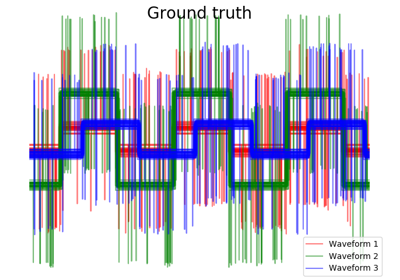# sklearn.metrics.pairwise_distances¶

sklearn.metrics.pairwise_distances(X, Y=None, metric='euclidean', n_jobs=None, **kwds)[source]

Compute the distance matrix from a vector array X and optional Y.

This method takes either a vector array or a distance matrix, and returns a distance matrix. If the input is a vector array, the distances are computed. If the input is a distances matrix, it is returned instead.

This method provides a safe way to take a distance matrix as input, while preserving compatibility with many other algorithms that take a vector array.

If Y is given (default is None), then the returned matrix is the pairwise distance between the arrays from both X and Y.

Valid values for metric are:

• From scikit-learn: [‘cityblock’, ‘cosine’, ‘euclidean’, ‘l1’, ‘l2’, ‘manhattan’]. These metrics support sparse matrix inputs.
• From scipy.spatial.distance: [‘braycurtis’, ‘canberra’, ‘chebyshev’, ‘correlation’, ‘dice’, ‘hamming’, ‘jaccard’, ‘kulsinski’, ‘mahalanobis’, ‘minkowski’, ‘rogerstanimoto’, ‘russellrao’, ‘seuclidean’, ‘sokalmichener’, ‘sokalsneath’, ‘sqeuclidean’, ‘yule’] See the documentation for scipy.spatial.distance for details on these metrics. These metrics do not support sparse matrix inputs.

Note that in the case of ‘cityblock’, ‘cosine’ and ‘euclidean’ (which are valid scipy.spatial.distance metrics), the scikit-learn implementation will be used, which is faster and has support for sparse matrices (except for ‘cityblock’). For a verbose description of the metrics from scikit-learn, see the __doc__ of the sklearn.pairwise.distance_metrics function.

Read more in the User Guide.

Parameters: X : array [n_samples_a, n_samples_a] if metric == “precomputed”, or, [n_samples_a, n_features] otherwise Array of pairwise distances between samples, or a feature array. Y : array [n_samples_b, n_features], optional An optional second feature array. Only allowed if metric != “precomputed”. metric : string, or callable The metric to use when calculating distance between instances in a feature array. If metric is a string, it must be one of the options allowed by scipy.spatial.distance.pdist for its metric parameter, or a metric listed in pairwise.PAIRWISE_DISTANCE_FUNCTIONS. If metric is “precomputed”, X is assumed to be a distance matrix. Alternatively, if metric is a callable function, it is called on each pair of instances (rows) and the resulting value recorded. The callable should take two arrays from X as input and return a value indicating the distance between them. n_jobs : int or None, optional (default=None) The number of jobs to use for the computation. This works by breaking down the pairwise matrix into n_jobs even slices and computing them in parallel. None means 1 unless in a joblib.parallel_backend context. -1 means using all processors. See Glossary for more details. **kwds : optional keyword parameters Any further parameters are passed directly to the distance function. If using a scipy.spatial.distance metric, the parameters are still metric dependent. See the scipy docs for usage examples. D : array [n_samples_a, n_samples_a] or [n_samples_a, n_samples_b] A distance matrix D such that D_{i, j} is the distance between the ith and jth vectors of the given matrix X, if Y is None. If Y is not None, then D_{i, j} is the distance between the ith array from X and the jth array from Y.

pairwise_distances_chunked
paired_distances
## Examples using sklearn.metrics.pairwise_distances¶